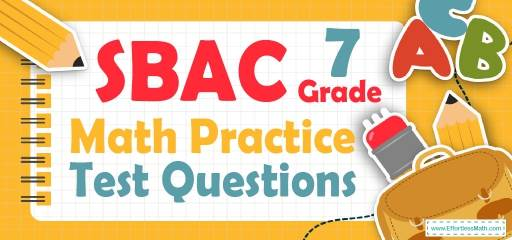# 7th Grade SBAC Math Practice Test Questions## 10 Sample 7th Grade SBAC Math Practice Questions

1- A chemical solution contains $$4\%$$ alcohol. If there is 24 ml of alcohol, what is the volume of the solution?

A. 240 ml

B. 480 ml

C. 600 ml

D. 1200 ml

2- The price of a laptop is decreased by $$10\%$$ to $360. What is its original price? A. 320 B. 380 C. 400 D. 450 3- What is the median of these numbers? $$4, 9, 13, 8, 15, 18, 5$$ A. 8 B. 9 C. 13 D. 15 4- Three times the price of a laptop is equal to five times the price of a computer. If the price of the laptop is$200 more than a computer, what is the price of the computer?

A. 300

B. 500

C. 800

D. 1500

5- What is the perimeter of a square that has an area of 595.36 feet? ________

6- Jason is 9 miles ahead of Joe running at 5.5 miles per hour and Joe is running at the speed of 7 miles per hour. How long does it take Joe to catch Jason?

A. 3 hours

B. 4 hours

C. 6 hours

D. 8 hours

7- 55 students took an exam and 11 of them failed. What percent of the students passed the exam?

A. $$20 \%$$

B. $$40 \%$$

C. $$60 \%$$

D. $$80 \%$$

8- Jason needs an $$75\%$$ average in his writing class to pass. On his first 4 exams, he earned scores of $$68\%, 72\%, 85\%$$, and $$90\%$$. What is the minimum score Jason can earn on his fifth and final test to pass? __________

9- A bank is offering $$3.5\%$$ simple interest on a savings account. If you deposit $12,000, how much interest will you earn in two years? A.$420

B. $840 C.$4200

D. $8400 10- 5 less than twice a positive integer is 83. What is the integer? A. 39 B. 41 C. 42 D. 44 ## Best 7th Grade SBAC Math Prep Resource for 2022 ## Answers: 1- C $$4\%$$ of the volume of the solution is alcohol. Let $$x$$ be the volume of the solution. Then: $$4\%$$ of $$x = 24$$ ml $$⇒ 0.04 x = 24 ⇒ x = 24 ÷ 0.04 = 600$$ 2- C Let $$x$$ be the original price. If the price of a laptop is decreased by $$10\%$$ to$360, then:
$$90 \%$$ of $$x=360 ⇒ 0.90x=360 ⇒ x=360÷0.90=400$$

3- B
Write the numbers in order:
$$4, 5, 8, 9, 13, 15, 18$$
Since we have 7 numbers (7 is odd), then the median is the number in the middle, which is 9.

4- A
Let $$L$$ be the price of laptop and $$C$$ be the price of computer.
$$3(L) =5(C) \space and \space L = 200 + C$$
Therefore, $$3(200 + C) =5C ⇒ 600 + 3C = 5C ⇒ C=300$$

5- 97.6
Use the area of square formula.
$$S = a^2 ⇒ 595.36 = a^2 ⇒ a = 24.4$$
Use the perimeter of square formula.
$$P = 4a ⇒ P=4(24.4) ⇒ P = 97.6$$

6- C
The distance between Jason and Joe is 9 miles. Jason running at 5.5 miles per hour and Joe is running at the speed of 7 miles per hour. Therefore, every hour the distance is 1.5 miles less.
$$9 ÷ 1.5 = 6$$

7- D
The failing rate is 11 out of $$55 = \frac{11}{55}$$.
Change the fraction to percent:
$$\frac{11}{55} ×100\%=20\%$$
20 percent of students failed. Therefore, 80 percent of students passed the exam.

8- 60
Jason needs an $$75\%$$ average to pass for five exams. Therefore, the sum of 5 exams must be at lease $$5 × 75 = 375$$
The sum of 4 exams is:
$$68 + 72 + 85 + 90 = 315$$
The minimum score Jason can earn on his fifth and final test to pass is:
$$375 – 315 = 60$$

9- B
Use simple interest formula:
$$I=prt$$
(I = interest, p = principal, r = rate, t = time)
$$I=(12000)(0.035)(2)=840$$

10- D
Let $$x$$ be the integer. Then:
$$2x – 5 = 83$$
Add 5 both sides: $$2x = 88$$
Divide both sides by 2: $$x = 44$$

Looking for the best resource to help you succeed on the SBAC Math Grade 7 Math test?

## The Best Books to Ace the 7th Grade SBAC Math Test

### What people say about "7th Grade SBAC Math Practice Test Questions - Effortless Math: We Help Students Learn to LOVE Mathematics"?

No one replied yet.

X
21% OFF

Limited time only!

Save Over 21%

SAVE $5 It was$23.99 now it is \$18.99